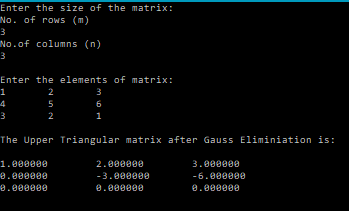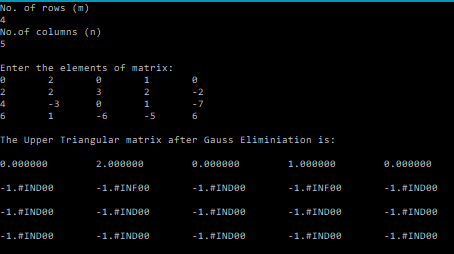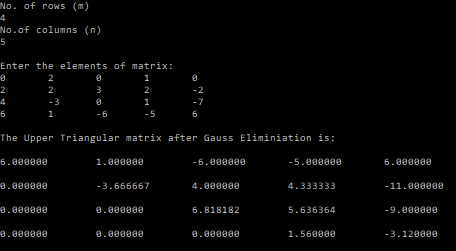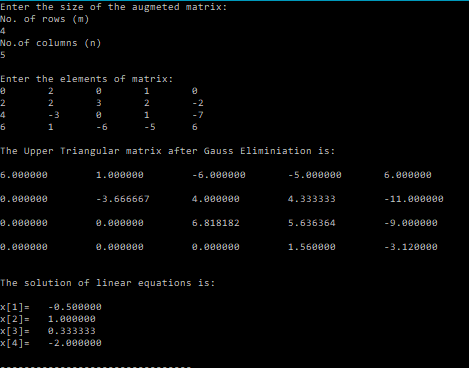# Gauss Elimination – C PROGRAM

In this post I am sharing with you, several versions of codes, which essentially perform Gauss elimination on a given matrix and reduce the matrix to the echelon form.
The following code performs Gauss Elimination on a given$m\times n$ matrix and reduces it to upper triangular matrix in echelon form.

### CODE (Without partial pivoting and back-substitution):

/**************************************************
****GAUSS ELIMINATION WITHOUT PARTIAL PIVOTING*****
**************************************************/
#include<stdio.h>
/*******
Function that performs Gauss-Elimination and returns the Upper triangular matrix:
There are two options to do this in C.
1. Pass a matrix (a) as the parameter, and calculate and store the upperTriangular(Gauss-Eliminated Matrix) in it.
2. Use malloc and make the function of pointer type and return the pointer.
This program uses the first option.
********/
double gaussElimination(int m, int n, double a[m][n]){
int i,j,k;
for(i=0;i<m-1;i++){
for(k=i+1;k<m;k++){
double  term=a[k][i]/a[i][i];
for(j=0;j<n;j++){
a[k][j]=a[k][j]-term*a[i][j];
}
}
}

}
/*******
Function that reads the elements of a matrix row-wise
Parameters: rows(m),columns(n),matrix[m][n]
*******/
void readMatrix(int m, int n, double matrix[m][n]){
int i,j;
for(i=0;i<m;i++){
for(j=0;j<n;j++){
scanf("%lf",&matrix[i][j]);
}
}
}
/*******
Function that prints the elements of a matrix row-wise
Parameters: rows(m),columns(n),matrix[m][n]
*******/
void printMatrix(int m, int n, double matrix[m][n]){
int i,j;
for(i=0;i<m;i++){
for(j=0;j<n;j++){
printf("%lf\t",matrix[i][j]);
}
printf("\n");
}
}
/*******
Function that copies the elements of a matrix to another matrix
Parameters: rows(m),columns(n),matrix1[m][n] , matrix2[m][n]
*******/
void copyMatrix(int m, int n, double matrix1[m][n], double matrix2[m][n]){
int i,j;
for(i=0;i<m;i++){
for(j=0;j<n;j++){
matrix2[i][j]=matrix1[i][j];
}
}
}

int main(){
int m,n,i,j;
printf("Enter the size of the matrix:\nNo. of rows (m)\n");
scanf("%d",&m);
printf("No.of columns (n)\n");
scanf("%d",&n);
//Declare a matrix to store the user given matrix
double a[m][n];
//Declare another matrix to store the resultant matrix obtained after Gauss Elimination
double U[m][n];
printf("\nEnter the elements of matrix:\n");
copyMatrix(m,n,a,U);
//Perform Gauss Elimination
gaussElimination(m,n,U);
printf("\nThe Upper Triangular matrix after Gauss Eliminiation is:\n\n");
printMatrix(m,n,U);

}


### OUTPUT:However, you will notice that this is not stable for all matrices.
Ex:As is evident here, the algorithm became unstable for the above example.

The stability of the program can be improved by employing partial pivoting.
So the following code implements that.

### CODE (With partial pivoting):

/**************************************************
*****GAUSS ELIMINATION WITH PARIAL PIVOTING********
**************************************************/
#include<stdio.h>
#include<math.h>
/*******
Function that performs Gauss-Elimination and returns the Upper triangular matrix:
There are two options to do this in C.
1. Pass a matrix (a) as the parameter, and calculate and store the upperTriangular(Gauss-Eliminated Matrix) in it.
2. Use malloc and make the function of pointer type and return the pointer.
This program uses the first option.
********/
void gaussElimination(int m, int n, double a[m][n]){
int i,j,k;
for(i=0;i<m-1;i++){
//Partial Pivoting
for(k=i+1;k<m;k++){
//If diagonal element(absolute vallue) is smaller than any of the terms below it
if(fabs(a[i][i])<fabs(a[k][i])){
//Swap the rows
for(j=0;j<n;j++){
double temp;
temp=a[i][j];
a[i][j]=a[k][j];
a[k][j]=temp;
}
}
}
//Begin Gauss Elimination
for(k=i+1;k<m;k++){
double  term=a[k][i]/ a[i][i];
for(j=0;j<n;j++){
a[k][j]=a[k][j]-term*a[i][j];
}
}
}

}
/*******
Function that reads the elements of a matrix row-wise
Parameters: rows(m),columns(n),matrix[m][n]
*******/
void readMatrix(int m, int n, double matrix[m][n]){
int i,j;
for(i=0;i<m;i++){
for(j=0;j<n;j++){
scanf("%lf",&matrix[i][j]);
}
}
}
/*******
Function that prints the elements of a matrix row-wise
Parameters: rows(m),columns(n),matrix[m][n]
*******/
void printMatrix(int m, int n, double matrix[m][n]){
int i,j;
for(i=0;i<m;i++){
for(j=0;j<n;j++){
printf("%lf\t",matrix[i][j]);
}
printf("\n");
}
}
/*******
Function that copies the elements of a matrix to another matrix
Parameters: rows(m),columns(n),matrix1[m][n] , matrix2[m][n]
*******/
void copyMatrix(int m, int n, double matrix1[m][n], double matrix2[m][n]){
int i,j;
for(i=0;i<m;i++){
for(j=0;j<n;j++){
matrix2[i][j]=matrix1[i][j];
}
}
}

int main(){
int m,n,i,j;
printf("Enter the size of the matrix:\nNo. of rows (m)\n");
scanf("%d",&m);
printf("No.of columns (n)\n");
scanf("%d",&n);
//Declare a matrix to store the user given matrix
double a[m][n];
//Declare another matrix to store the resultant matrix obtained after Gauss Elimination
double U[m][n];
printf("\nEnter the elements of matrix:\n");
copyMatrix(m,n,a,U);
//Perform Gauss Elimination
gaussElimination(m,n,U);
printf("\nThe Upper Triangular matrix after Gauss Eliminiation is:\n\n");
printMatrix(m,n,U);

}


### OUTPUT:

This time when you run the previous example, you will see that the program still works.The Gauss elimination technique can be used to solve a system of linear equations, by asking the user to input an augmented matrix(Wikipedia) that contains the coefficients as well as the RHS of the equations.
This can be done by adding a small back-substitution procedure.
The following code solves a system of equations using Gauss elimination and back-substitution.
NOTE: The code is compatible with the number of equations being more than the number of variables. However, the number of variables can’t/shouldn’t be less than the number of equations.

### CODE (With back-substitution):

/**************************************************
*****SOLVING SYSTEM OF LINEAR EQUATIONS WITH*******
*****GAUSS ELIMINATION WITH PARIAL PIVOTING********
**************************************************/
#include<stdio.h>
#include<math.h>
/*******
Function that performs Gauss-Elimination and returns the Upper triangular matrix and solution of equations:
There are two options to do this in C.
1. Pass the augmented matrix (a) as the parameter, and calculate and store the upperTriangular(Gauss-Eliminated Matrix) in it.
2. Use malloc and make the function of pointer type and return the pointer.
This program uses the first option.
********/
void gaussEliminationLS(int m, int n, double a[m][n], double x[n-1]){
int i,j,k;
for(i=0;i<m-1;i++){
//Partial Pivoting
for(k=i+1;k<m;k++){
//If diagonal element(absolute vallue) is smaller than any of the terms below it
if(fabs(a[i][i])<fabs(a[k][i])){
//Swap the rows
for(j=0;j<n;j++){
double temp;
temp=a[i][j];
a[i][j]=a[k][j];
a[k][j]=temp;
}
}
}
//Begin Gauss Elimination
for(k=i+1;k<m;k++){
double  term=a[k][i]/ a[i][i];
for(j=0;j<n;j++){
a[k][j]=a[k][j]-term*a[i][j];
}
}

}
//Begin Back-substitution
for(i=m-1;i>=0;i--){
x[i]=a[i][n-1];
for(j=i+1;j<n-1;j++){
x[i]=x[i]-a[i][j]*x[j];
}
x[i]=x[i]/a[i][i];
}

}
/*******
Function that reads the elements of a matrix row-wise
Parameters: rows(m),columns(n),matrix[m][n]
*******/
void readMatrix(int m, int n, double matrix[m][n]){
int i,j;
for(i=0;i<m;i++){
for(j=0;j<n;j++){
scanf("%lf",&matrix[i][j]);
}
}
}
/*******
Function that prints the elements of a matrix row-wise
Parameters: rows(m),columns(n),matrix[m][n]
*******/
void printMatrix(int m, int n, double matrix[m][n]){
int i,j;
for(i=0;i<m;i++){
for(j=0;j<n;j++){
printf("%lf\t",matrix[i][j]);
}
printf("\n");
}
}
/*******
Function that copies the elements of a matrix to another matrix
Parameters: rows(m),columns(n),matrix1[m][n] , matrix2[m][n]
*******/
void copyMatrix(int m, int n, double matrix1[m][n], double matrix2[m][n]){
int i,j;
for(i=0;i<m;i++){
for(j=0;j<n;j++){
matrix2[i][j]=matrix1[i][j];
}
}
}

int main(){
int m,n,i,j;
printf("Enter the size of the augmeted matrix:\nNo. of rows (m)\n");
scanf("%d",&m);
printf("No.of columns (n)\n");
scanf("%d",&n);
//Declare a matrix to store the user given matrix
double a[m][n];
//Declare another matrix to store the resultant matrix obtained after Gauss Elimination
double U[m][n];
//Declare an array to store the solution of equations
double x[m];
printf("\nEnter the elements of matrix:\n");
copyMatrix(m,n,a,U);
//Perform Gauss Elimination
gaussEliminationLS(m,n,U,x);
printf("\nThe Upper Triangular matrix after Gauss Eliminiation is:\n\n");
printMatrix(m,n,U);
printf("\nThe solution of linear equations is:\n\n");
for(i=0;i<n-1;i++){
printf("x[%d]=\t%lf\n",i+1,x[i]);
}
}


### OUTPUT:### Android Apps:

I’ve also created a few Android apps that perform various matrix operations and can come in handy to those taking a course on Numerical Methods.

### References:

Well, that’s it!
I hope you guys enjoyed this post.

If you have any questions/doubts leave them in the comments section down below.[wpedon id="7041" align="center"]

## One thought on “Gauss Elimination – C PROGRAM”

1. Is it true that your code (with back-substitution) performs the following count of floating point operations on nxn matrix and n variables?

multiply — n^2